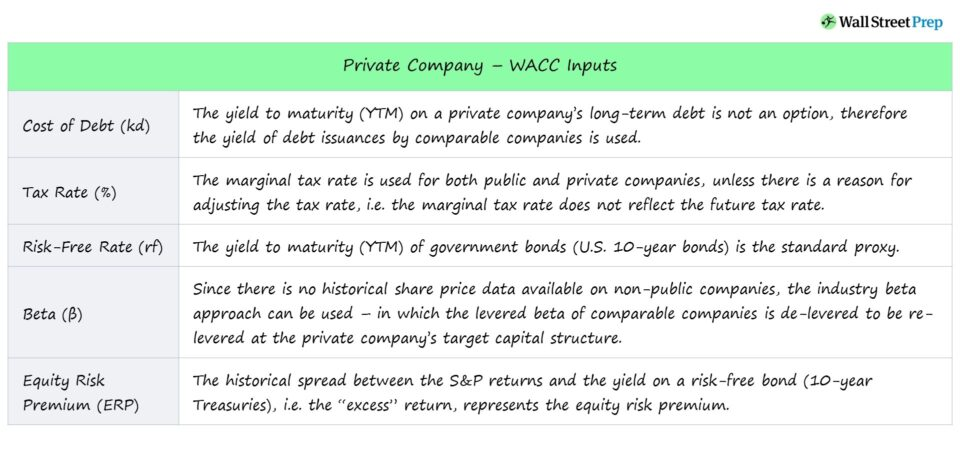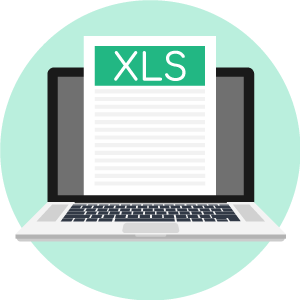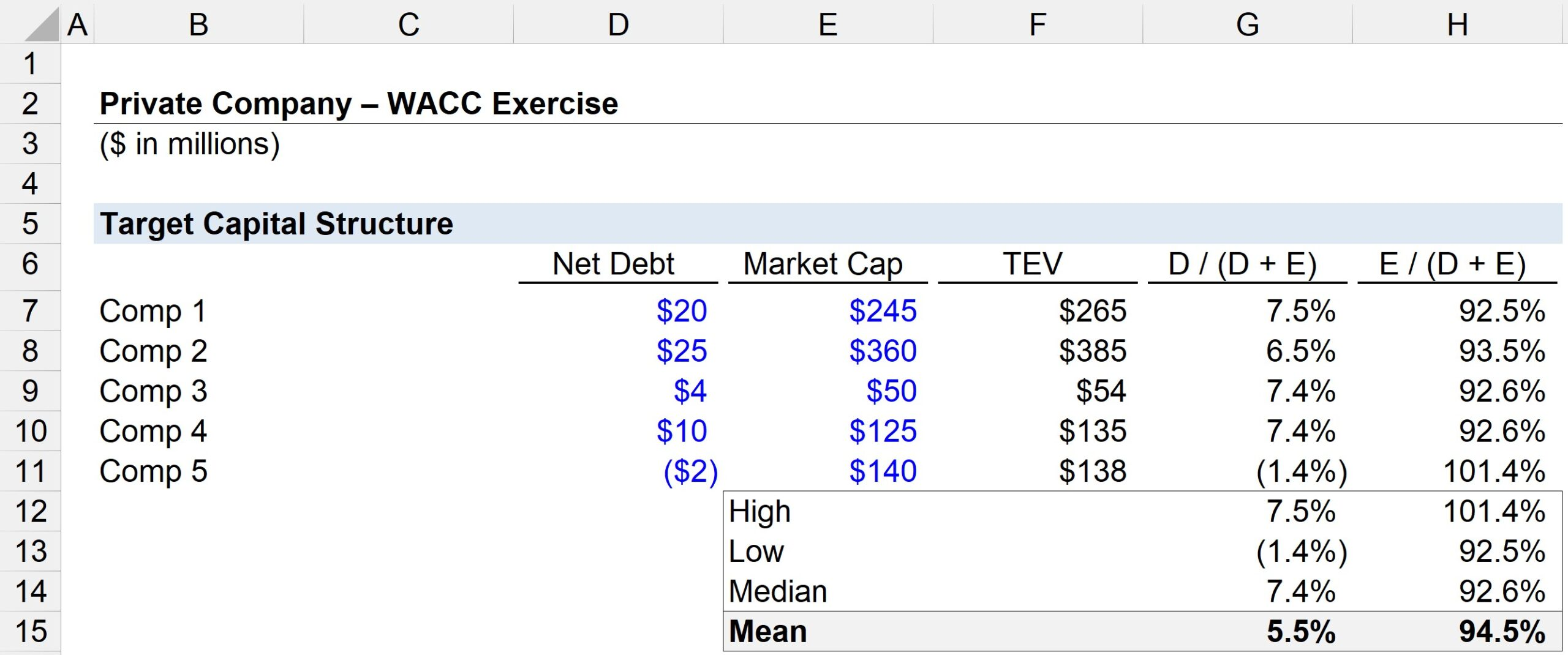# WACC for a Private Company

Guide to Understanding WACC for a Private Company• How is the WACC calculated for a private company?
• How is the calculation of WACC for a private company different from a public company?
• What method estimates a private company’s target capital structure?
• What are the steps to the industry beta approach?

## Introduction to Private Companies

A private company refers to a company not currently traded on the public markets.

Like public companies, private companies also issue shares, but the distinction is that those shares are not traded on a stock exchange.

In fact, the majority of businesses in the U.S. are privately held, which include early-stage startups, mom-and-pop shops, small and medium-sized businesses (SMBs), and companies with backing from growth equity firms on the cusp of going public via IPO (or a direct listing).

Contrary to a common misconception, not all private companies are early-stage and/or are yet to gain traction, and private companies can vary widely in terms of their phase of maturity.

###### Drawbacks to IPOs (“Going Public”)

Nowadays, more companies are opting to remain private for longer because of the downsides of going public, such as:

• Public Filing Requirements (SEC)
• Standardized Financial Reporting (GAAP/IFRS)
• Regulatory Implications
• External Shareholders
• Dilution of Equity Ownership

## Private Companies vs Public Companies Differences

The main difference between valuing a private and public company is the availability of data and disclosures.

The limited availability of a non-public company’s financial data, corporate governance, and business strategy can make valuing private companies challenging.

Unlike public companies, private companies are under no obligation to make their financial statements public.

If you’re provided with the financials of a private company, the valuation process itself is similar to that of public companies, except the financial disclosures of private companies are not standardized (and thus, not as reliable).

Public companies in the U.S. must abide by strict accounting policies in accordance with U.S. GAAP and filing requirements established by the SEC (e.g. 10-Q, 10-K) – not to mention, regular audits by accounting firms.

In contrast, private companies have far more individual discretion – i.e. less regulatory oversight – when it comes to recording their financials and other disclosures.

In practice, the limited availability of information and the absence of standardization creates additional steps to the valuation of a private company, such as the following:

• Normalizing Cash Flows and Fixing Inconsistencies (or Spotty) Data
• Modifying Compensation of Management (i.e. More Consistent to Market-Rate)
• Adjusting Financial Statements Structure Closer to GAAP

## Intrinsic Value of Private Company – DCF Challenges

The process of valuing a private company is not all that different from the methods utilized to value public companies.

Often, a discounted cash flow (DCF) analysis is used to estimate the intrinsic value of a private company.

1. The future free cash flows (FCFs) generated by the company are forecasted/projected.
2. The FCFs are discounted to the present date using an appropriate discount rate (WACC) that reflects the riskiness of the cash flows.
3. The sum of the discounted cash flows – including the explicit forecast period and the terminal value – represent the company’s present value (PV), i.e. the enterprise value.

However, the following challenges emerge from attempting to determine the intrinsic value of a private company.

• Equity Value: The equity value of the private companies, or “market capitalization”, cannot be calculated, as there is no readily available market share price to multiply the total shares outstanding by.
• Optimal Capital Structure: The target capital structure of a private company is less straightforward as the cost of equity and cost of debt will be higher for a private company than for a comparable public counterpart.
• Market Value of Debt: Like the equity value, the market value of the private company’s debt is also not available, nor is there typically a publicly available yield to maturity (YTM) of its debt issuances.
• Illiquidity Discount: Private companies are less liquid – i.e. there is less marketability and buyers to sell shares too – and should thus their valuation should reflect an illiquidity discount, a reduction in value that typically ranges between ~10% to 30%.

## Private Company WACC – Discount Rate Calculation

The weighted average cost of capital (WACC) is the discount rate used to discount unlevered free cash flows (i.e. free cash flow to the firm), as all capital providers are represented.

The WACC formula consists of multiplying the after-tax cost of debt by the debt weight, which is then added to the product of the cost of equity and the equity weight.

###### Weighted Average Cost of Capital (WACC) Formula
• WACC = [After-Tax Cost of Debt * (Debt / (Debt + Equity)] + [Cost of Equity * (Equity / (Debt + Equity)]

The considerations when calculating the WACC for a private company are as follows:

• Cost of Debt (rd): The yield to maturity (YTM) on a private company’s long term debt is not typically publicly available, therefore the yield of debt issuances by comparable companies (i.e. comparable public companies with similar credit ratings) is used – with the data sourced from credit agencies (Moody’s, S&P).
• Tax Rate (%): The marginal tax rate is used for both public and private companies unless there is a reason for adjusting the tax rate, i.e. the marginal tax rate does not reflect the future tax rate.
• Risk-Free Rate (rf): The yield to maturity (YTM) of government bonds, most often the U.S. 10-year bonds) is the standard proxy for the risk-free rate.
• Beta (β): Since there is no historical share price data available on a non-public company, running a regression model against the broader market returns is not an option – thus, the industry beta can be used, in which the levered beta of comparable companies is de-levered to be re-levered at the target capital structure of the private company.
• Equity Risk Premium (ERP): The historical spread between the S&P returns and the yield on a risk-free bond (10-year Treasuries), i.e. the “excess” market return, represents the equity risk premium.

## WACC Inputs for a Private Company

The lesson below provides a concise summarization of the WACC inputs for private companies:

Source: The Practitioner’s Guide to Private Company Analysis Course

## Private Company WACC Exercises – Excel Template

We’ll now move to a modeling exercise, which you can access by filling out the form below.Submitting ...

## Target Capital Structure Calculation

Suppose we are attempting to calculate the WACC of a private company, but there are two issues we have encountered:

1. What should the equity and debt component weights (%) in the private company’s target capital structure be?
2. What is the private company’s beta?

In order to figure out the answer to those two inputs (and obtain the other inputs necessary to the WACC formula), we have gathered the data on five comparable companies.

Net Debt Market Cap Enterprise Value (TEV)
Comp 1 \$20 million \$245 million \$265 million
Comp 2 \$25 million \$360 million \$385 million
Comp 3 \$4 million \$50 million \$54 million
Comp 4 \$10 million \$125 million \$135 million
Comp 5 (\$2) million \$140 million \$138 million

Using these assumptions, we can estimate the target capital structure for our company.

The first step is to calculate the debt weight of each comp – i.e. Debt-to-Capital – which is equal to the net debt amount divided by the sum of the net debt and equity value.

###### Capitalization Ratio
• Debt-to-Equity Ratio = Total Debt / (Total Debt + Total Equity)

Either gross debt or net debt can be used, but we will be using net debt for our example – the intuition being that the cash on the balance sheet could hypothetically be used to pay off some of the existing debt.

In the next step, we calculate the equity weight of each comp by dividing the equity value by the total capitalization. (or we could subtract the debt weight from one).

###### Comps Analysis – Excel Functions Review

With those two columns done, we’ll now assess the results using the following Excel functions.

• High =”MAX” Function
• Low =”MIN” Function
• Median = “MEDIAN” Function
• Mean =”AVERAGE” Function

Upon completion of all calculations, we arrive at the following average (i.e. the mean) debt and equity weights, which we reference in the WACC of the private company.

• Median → Debt Weight = 7.4%; Equity Weight = 92.6%
• Mean → Debt Weight =5.5%; Equity Weight = 94.5%## Beta Calculation (De-Levered to Re-Levered β)

In the next part of our modeling exercise, we will calculate the industry beta (β), which requires us to de-lever and then re-lever beta at our company’s target capital structure.

Observed Beta Tax Rate (%)
Comp 1 0.25 25.0%
Comp 2 0.60 18.0%
Comp 3 0.45 26.0%
Comp 4 0.50 21.0%
Comp 5 0.60 24.0%

Before calculating the de-levered beta, we must first pull the market cap and net debt values from the prior section.

We can then calculate the debt-to-equity ratio (D/E), which we’ll need later.

As confirmed by the varying capital structures of our peer group, we must remove the distorting impact of different D/E ratios on beta.

The more leverage carried by a company, the higher its observed β will be – all being else equal – as interest payments cause cash flows to equity holders to be more volatile.

In order to calculate the de-levered beta for each comp, the equation shown below is used.

###### De-Levered β Formula
• De-Levered β = Observed β / [1 + (1 – Tax Rate) * D/E Ratio)

After each comp’s de-levered beta is calculated, we can calculate the industry average de-levered beta, which comes out to 0.46.

In the final step, we can now re-lever beta at the target capital structure.

For purposes of simplicity, the marginal tax rate will be assumed to be the average of all the comparable companies’ tax rates, while the target net debt/equity will also be assumed to be the average D/E ratio.

• Marginal Tax Rate =22.8%
• Net Debt / Equity Ratio =5.9%

The re-levered beta of our private company comes out to 0.48, as shown below.

• Re-Levered β = 0.46 * (1 + (1 – 22.8%) * 5.9%)
• Re-Levered β = 0.48

In our illustrative modeling exercise, we calculated our private company’s target capital structure and beta, two critical inputs to the WACC formula, using the industry beta / comparable companies approach.Step-by-Step Online Course

#### Everything You Need To Master Financial Modeling

Enroll in The Premium Package: Learn Financial Statement Modeling, DCF, M&A, LBO and Comps. The same training program used at top investment banks.Inline FeedbacksLearn Financial Modeling Online

Everything you need to master financial and valuation modeling: 3-Statement Modeling, DCF, Comps, M&A and LBO.

X

The Wall Street Prep Quicklesson Series

7 Free Financial Modeling Lessons

Get instant access to video lessons taught by experienced investment bankers. Learn financial statement modeling, DCF, M&A, LBO, Comps and Excel shortcuts.